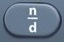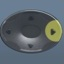# Rectangle

Rectangle = Flat Shape with Four Sides (with four right angles)

#Basics

l = Length
w = Width
P = Perimeter = distance along sides of rectangle

A = Area = area covering surface of rectangle (measured in square units)
Right Angle = 90°

A rectangle has four right angles.
The opposite sides of a rectangle are parallel.
A rectangle is a special form of a parallelogram in which all angles are right angles.

Formulas (Mathematics Formula Sheet)

P = 2l + 2w

A = lw

Formulas (NOT on Mathematics Formula Sheet)
l =$\bf\displaystyle\frac{P-2w}{2}$

w =$\bf\displaystyle \frac{P-2l}{2}$

l = A ÷ w

w = A ÷ l

Question
A football field is 120 yards long and 53 yards wide.  What is its perimeter?

346 yards

P = 2l + 2w
P = 2 × 120 + 2 × 53 = 346

Input Display Commentblinker clears screen
2 × 120 + 2 × 53 2*120+2*53 P = 2l + 2w346 Answer
###### Rectangle

Question
A football field is 120 yards long and 53 yards wide.  What is its area?

6360 square yards

A = lw
A = 120 × 53 = 6360

Input Display Commentblinker clears screen
120 × 53 120*53 A = lw6360 Answer
###### Rectangle

Question
The perimeter of a rectangular ping pong table is 8.5 meters.  Its width is 1.5 meters.  What is its length?

2.75 meters

l =$\bf\displaystyle\frac{P-2w}{2}$

l =$\bf\displaystyle\frac{8.5-2\hspace{0.1cm}x\hspace{0.1cm}1.5}{2}$ = 2.75

Input Display Commentblinker clears screen8.5-2×1.52$\bf\displaystyle\frac{8.5-2*1.5}{2}$ l =$\bf\displaystyle\frac{P-2w}{2}$2.75 Answer
###### Rectangle

Question
The perimeter of a rectangular candy bar is 14 centimeters.  Its length is 5 centimeters.  What is its width?

2 centimeters

w =$\bf\displaystyle \frac{P-2l}{2}$

w =$\bf\displaystyle\frac{14-2\hspace{0.1cm}x\hspace{0.1cm}5}{2}$ = 2

Input Display Commentblinker clears screen14-2×52$\bf\displaystyle\frac{14-2*5}{2}$ w =$\bf\displaystyle \frac{P-2l}{2}$2 Answer
###### Rectangle

Question
The area of a rectangular room is 108 square feet.  Its width is 9 feet.  What is its length?

12 feet

l = A ÷ w
l = 108 ÷ 9 = 12

Input Display Commentblinker clears screen
108 ÷ 9 108÷9 l = A ÷ w12 Answer
###### Rectangle

Question

The area of a rectangular room is 156 square feet.  Its length is 13 feet.  What is its width?

12 feet

w = A ÷ l
w = 156 ÷ 13 = 12

Input Display Commentblinker clears screen
156 ÷ 13 156÷13 w = A ÷ l12 Answer
###### Rectangle

Question
The area of a rectangular postage stamp is 9.72 square centimeters.  Its width is 2.7 centimeters.  What is its perimeter?

12.6 centimeters

l = A ÷ w
l = 9.72 ÷ 2.7 = 3.6

P = 2l + 2w
P = 2 × 3.6  +  2 × 2.7 = 12.6

Input Display Commentblinker clears screen
9.72 ÷ 2.7 9.72÷2.7 l = A ÷ w3.6 l
2 × 3.6 + 2 × 2.7 2*3.6+2*2.7 P = 2l + 2w12.6 Answer
###### Rectangle

Question
The area of a rectangular postage stamp is 10.92 square centimeters.  Its length is 3.9 centimeters.  What is its perimeter?

13.4 centimeters

w = A ÷ l
w = 10.92 ÷ 3.9 = 2.8

P = 2l + 2w
P = 2 × 3.9  +  2 × 2.8 = 13.4

Input Display Commentblinker clears screen
10.92 ÷ 3.9 10.92÷3.9 w = A ÷ l2.8 w
2 × 3.9 + 2 × 2.8 2*3.9+2*2.8 P = 2l + 2w13.4 Answer
###### Rectangle

Question
The perimeter of a rectangular postage stamp is 20 centimeters.  Its width is 3 centimeters.  What is its area?

21 square centimeters

l =$\bf\displaystyle\frac{P-2w}{2}$

l =$\bf\displaystyle\frac{20-2\hspace{0.1cm}x\hspace{0.1cm}3}{2}$ = 7

A = lw
A = 7 × 3 = 21

Input Display Commentblinker clears screen20-2×32$\bf\displaystyle\frac{20-2\hspace{0.1cm}x\hspace{0.1cm}3}{2}$ l =$\bf\displaystyle\frac{P-2w}{2}$7 l
7 × 3 7*3 A = lw21 Answer
###### Rectangle

Question
The perimeter of a rectangular postage stamp is 24 centimeters.  Its length is 8 centimeters.  What is its area?

32 square centimeters

w =$\bf\displaystyle \frac{P-2l}{2}$

w =$\bf\displaystyle\frac{24-2\hspace{0.1cm}x\hspace{0.1cm}8}{2}$ = 4

A = lw
A = 8 × 4 = 32

Input Display Commentblinker clears screen24-2×82$\bf\displaystyle\frac{24-2*8}{2}$ w =$\bf\displaystyle \frac{P-2l}{2}$4 w
8 × 4 8*4 A = lw32 Answer
###### Rectangle

Practice – Questions
1.  A park is 525 yards long and 125 yards wide.  What is its perimeter?

2.  A park is 525 yards long and 125 yards wide.  What is its area?

3.  The perimeter of a large rectangular table is 12.5 meters.  Its width is 3.5 meters.  What is its length?

4.  The perimeter of a large rectangular candy bar is 22 centimeters.  Its length is 7 centimeters.  What is its width?

5.  The area of a rectangular room is 88 square feet.  Its width is 8 feet.  What is its length?

6.  The area of a rectangular room is 132 square feet.  Its length is 12 feet.  What is its width?

7.  The area of a rectangular tile is 35 square inches.  Its width is 5 inches.  What is its perimeter?

8.  The area of a rectangular tile is 64.66 square inches.  Its length is 12.2 inches.  What is its perimeter?

9.  The perimeter of a rectangular tile is 40 inches.  Its width is 8 inches.  What is its area?

10.  The perimeter of a large rectangular tile is 107.5 inches.  Its length is 12.5 inches.  What is its area?

1.  1300 yards

2.  65625 square yards

3.  2.75 meters

4.  4 centimeters

5.  11 feet

6.  11 feet

7.  24 inches

8.  35 inches

9.  96 square inches

10.  515.625 square inches